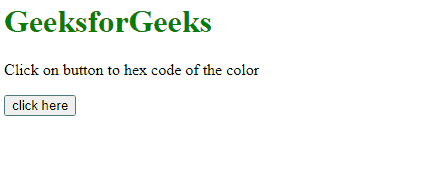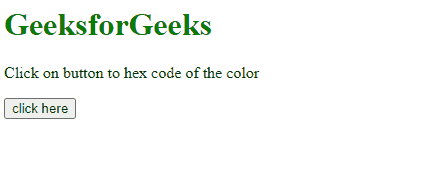# How to get hex color value of RGB value ?

Given the RGB color value and the task is to get the Hex Code of that color using JavaScript.

Approach 1:

• Call the convert() user-defined function and use RGB value as a parameter.
• Use the match() method to select the value of Red, Green, and Blue. The value of RGB is stored in the form of array.
• The hexCode() function call to convert the value of RGB to hexCode.
• The slice() method gets the part of a string and returns the extracted parts in a new string. The toString() method converts the number to string.

Example 1: This example gets the RGB value of white which is rgb(255,255,255) and converts it into HexCode by using JavaScript.

## html

 `<``h1` `style``=``"color:green;"``> ` `    ``GeeksforGeeks ` ` ` ` `  `<``p` `id``=``"GFG_UP"``> ` ` ` ` `  `<``button` `onClick``=``"GFG_Fun()"``> ` `    ``click here ` ` ` ` `  `<``p` `id``=``"GFG_DOWN"``> ` ` ` ` `  `<``script``> ` `    ``var up = document.getElementById('GFG_UP'); ` `    ``var down = document.getElementById('GFG_DOWN'); ` `    ``up.innerHTML = 'Click on button to hex code of the color'; ` `     `  `    ``function convert(rgb) { ` `        ``rgb = rgb.match(/^rgb\((\d+),\s*(\d+),\s*(\d+)\)\$/); ` `     `  `        ``function hexCode(i) { ` `            ``return ("0" + parseInt(i).toString(16)).slice(-2); ` `        ``} ` `        ``return "#" + hexCode(rgb) + hexCode(rgb) ` `                ``+ hexCode(rgb); ` `    ``} ` `     `  `    ``function GFG_Fun() { ` `        ``down.innerHTML = "Hex Code of 'white' = " ` `            ``+ convert('rgb(255,255,255)'); ` `    ``} ` ``

Output:How to get hex color value of RGB value ?

Example 2: This methods first checks, if hex value is provided instead of RGB value. Because some browsers return the hex value in place of the RGB value. This example gets the RGB value of green which is RGB(0,128,0) and converts it into HexCode by creating a function.

## html

 `<``h1` `style``=``"color:green;"``> ` `    ``GeeksforGeeks ` ` ` ` `  `<``p` `id``=``"GFG_UP"``> ` ` ` ` `  `<``button` `onClick``=``"GFG_Fun()"``> ` `    ``click here ` ` ` ` `  `<``p` `id``=``"GFG_DOWN"``> ` ` `  `<``script``> ` `    ``var up = document.getElementById('GFG_UP'); ` `    ``var down = document.getElementById('GFG_DOWN'); ` `    ``up.innerHTML = 'Click on button to hex code of the color'; ` `     `  `    ``function convert(rgb) { ` `        ``if (/^#[0-9A-F]{6}\$/i.test(rgb)) return rgb; ` `     `  `        ``rgb = rgb.match(/^rgb\((\d+),\s*(\d+),\s*(\d+)\)\$/); ` `     `  `        ``function hexCode(i) { ` `            ``return ("0" + parseInt(i).toString(16)).slice(-2); ` `        ``} ` `        ``return "#" + hexCode(rgb) + hexCode(rgb) ` `                ``+ hexCode(rgb); ` `    ``} ` `     `  `    ``function GFG_Fun() { ` `        ``down.innerHTML = "Hex Code of 'Green' = " ` `                ``+ convert('rgb(0,128,0)'); ` `    ``} ` ``

Output:How to get hex color value of RGB value ?

Whether you're preparing for your first job interview or aiming to upskill in this ever-evolving tech landscape, GeeksforGeeks Courses are your key to success. We provide top-quality content at affordable prices, all geared towards accelerating your growth in a time-bound manner. Join the millions we've already empowered, and we're here to do the same for you. Don't miss out - check it out now!